# Odd holes and odd walks

Given a graph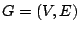and two vertices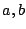can the following problem be solved in polynomial time ?

Find a triangle-free odd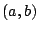-walk in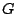, where a walk can also contain repetitions of edges, and triangle-free means that the vertex-set of the walk does not contain any triangle (but can contain an odd hole).

A polynomial algorithm for this problem would specialize to a polynomial algorithm for finding odd holes (and even pairs in odd-hole-free graphs). Bienstock proved that it is NP-hard to find odd holes containing a given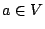. However, a triangle-free odd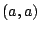-walk exists in a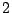-connected graph if and only if there exists an odd hole in(not necessarily containing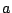).

Contributed by András Sebo and Nicolas Trotignon

Back to the main index for Perfect Graphs.